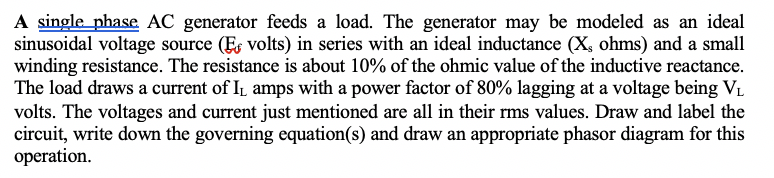# Question A single phase AC generator feeds a load. The generator may be modeled as an ideal sinusoidal voltage source (Ex volts) in series with an ideal inductance (X, ohms) and a small winding resistance. The resistance is about 10% of the ohmic value of the inductive reactance. The load draws a current of I, amps with a power factor of 80% lagging at a voltage being VL volts. The voltages and current just mentioned are all in their rms values. Draw and label the circuit, write down the governing equation(s) and draw an appropriate phasor diagram for this operation.CW7XUD The Asker · Electrical EngineeringTranscribed Image Text: A single phase AC generator feeds a load. The generator may be modeled as an ideal sinusoidal voltage source (Ex volts) in series with an ideal inductance (X, ohms) and a small winding resistance. The resistance is about 10% of the ohmic value of the inductive reactance. The load draws a current of I, amps with a power factor of 80% lagging at a voltage being VL volts. The voltages and current just mentioned are all in their rms values. Draw and label the circuit, write down the governing equation(s) and draw an appropriate phasor diagram for this operation.
More
Transcribed Image Text: A single phase AC generator feeds a load. The generator may be modeled as an ideal sinusoidal voltage source (Ex volts) in series with an ideal inductance (X, ohms) and a small winding resistance. The resistance is about 10% of the ohmic value of the inductive reactance. The load draws a current of I, amps with a power factor of 80% lagging at a voltage being VL volts. The voltages and current just mentioned are all in their rms values. Draw and label the circuit, write down the governing equation(s) and draw an appropriate phasor diagram for this operation.